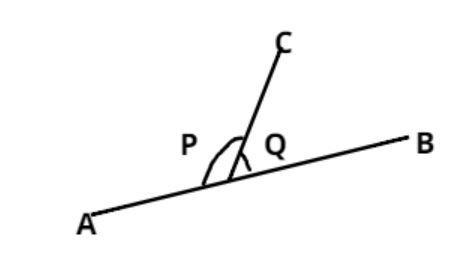Courses
Courses for Kids
Free study material
Offline Centres
MoreLast updated date: 03rd Dec 2023
Total views: 384.9k
Views today: 4.84k

# If two angles are supplementary, then their sum is${\text{A}}{\text{. 9}}{{\text{0}}^0} \\ {\text{B}}{\text{. 18}}{{\text{0}}^0} \\ {\text{C}}{\text{. 27}}{{\text{0}}^0} \\ {\text{D}}{\text{. 36}}{{\text{0}}^0} \\$VerifiedAs we know the condition of supplementary angle which is, two angle make a supplementary angle if the sum of two angles is ${180^0}$.
$\Rightarrow \angle P + \angle Q = {180^0}$(on a straight line the sum of angle is ${180^0}$)
Note – In such types of questions always recall the property of supplementary angle which is that the sum of two angles always make ${180^0}$, or we can also say that two right angle also make a supplementary angle, and we can also say that two supplementary angle always form a straight line.